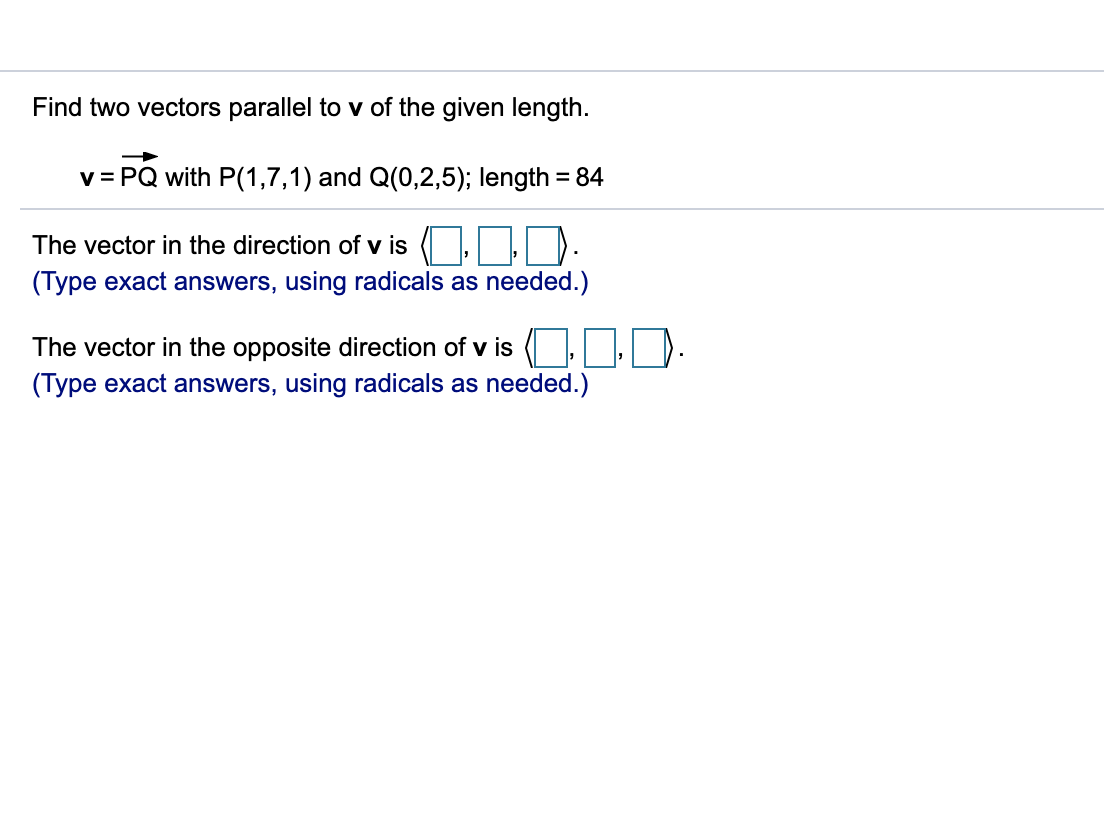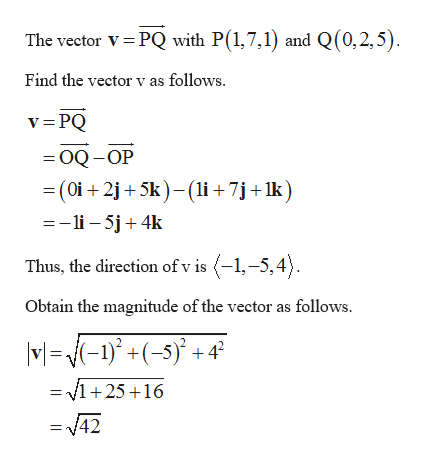# Find two vectors parallel to v of the given length.v PQ with P(1,7,1) and Q(0,2,5); length 84The vector in the direction of v is(Type exact answers, using radicals as needed.)The vector in the opposite direction of v is(Type exact answers, using radicals as needed.)

Question
83 viewshelp_outlineImage TranscriptioncloseFind two vectors parallel to v of the given length. v PQ with P(1,7,1) and Q(0,2,5); length 84 The vector in the direction of v is (Type exact answers, using radicals as needed.) The vector in the opposite direction of v is (Type exact answers, using radicals as needed.) fullscreen
check_circle

Step 1

From the given information, two vectors p...help_outlineImage TranscriptioncloseThe vector V PQ with P(1,7,1) and Q(0,2,5) Find the vector v as follows v=PQ OQ-OP (0i 2j5k-(li +7j +1k =-li-5j 4k Thus, the direction of v is (-1,-5,4) Obtain the magnitude of the vector as follows. M-)5) = v12516 = 42 fullscreen

### Want to see the full answer?

See Solution

#### Want to see this answer and more?

Solutions are written by subject experts who are available 24/7. Questions are typically answered within 1 hour.*

See Solution
*Response times may vary by subject and question.
Tagged in

### Calculus